Previous worksheets showed how to calculate the difference between different dates and different times. This worksheet expands on those by showing you how to calculate the difference (in hours) between different times on different dates. This could tell you how long someone had spent travelling etc.

You can then use this information directly in analyses, or use a further derived variable to break it down into ranges defined by time intervals (for example, short trip, medium trip, and long waits).

### Background

Snap stores date and time data in a format that you can use to make calculations. If you tell Snap that responses are a date or a time, Snap will use special calculations to work out the differences between them. The differences between dates are given in days, and the differences in times are given in seconds.

This example uses a journey starting at 9pm on Monday 1st and ending at 10am on Thursday 4th, and shows how to calculate the journey length.

By using two different types of derived variable, you can present the same data in quantitive and qualititive form, allowing for different types of analyses.

### Step 1: Creating the time and date questions

1. Open your questionnaire. This assumes that you are asking about someone’s journey to a holiday destination.
2. Enter the start date question.
• Create a question by clickingon the toolbar
• Set the style to Open First.
• Set the response type to Date.• Enter the question text “Please enter the date and time that you left home”.
• Enter the grid label text “Date of leaving”.
3. Enter the start time question
• Press the [Enter] key to create a new question.
• Set the style to Open Next.
• Set the response type to Time.
• Enter the grid label text “Time of leaving”.
4. Repeat for the arrival date and time questions, changing the text as appropriate.### Step 2: Changing the names of your variables for ease of calculation

The variables you have created will have the question numbers by default. Since we are going to use them in a formula, it is easier to give them a name that explains what they are for.

1. Right-click on your question asking for the “Date of leaving”, and select Variable properties from the context menu.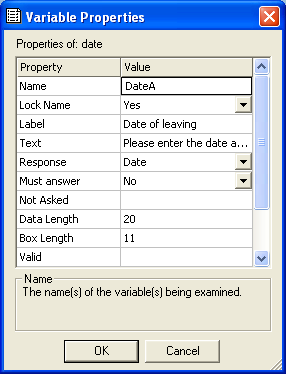2. Edit the name of the variable to DateA and click [OK].
3. Change the variable name for the time of leaving to TimeA in the same way.
4. Change the names of the arrival dates and times to DateB and TimeB.

### Step 3: Creating the derived variable to calculate the difference

You must now set up a derived variable for Snap to calculate the difference between the time when the respondent left home and their arrival time.

1. Click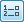to open the Variables window.
2. Clickto create a new variable.
3. Set the Name to timedelay, the Type to Derived and the response to Quantity.
4. Set a sensible Label (e.g, “Difference between leaving and arrival time in hours”)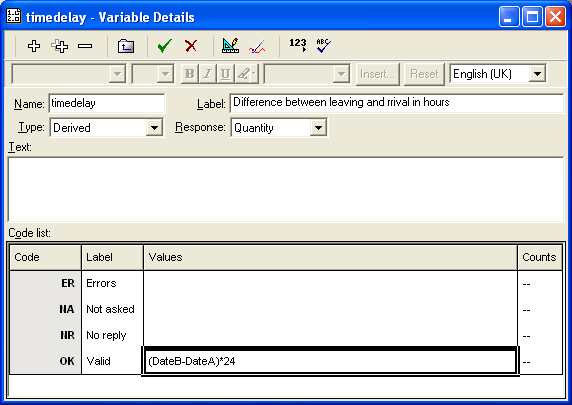5. Set up the formula in the Valid field.
6. The first part of the formula is working out the days spent on the journey and converting it to hours by multiplying it by 24.
`(DateB-DateA)*24`
7. The second part of the formula is the difference between the two times. If you subtract one time from another in Snap, Snap always give you a positive value. If the second time is before the first, Snap assumes that one must be before midnight and one must be after midnight, and works out a time across midnight. This means that 10am – 9pm gives 13 hours rather than -11 hours. Snap works out time differences in seconds. To convert the time difference to hours, you must divide it by 3600
`(TimeB-TimeA)/3600````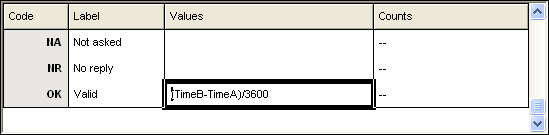```
8. The last part of the formula is to sort out what happens if the second time was before the first. The diagram below shows a journey starting at 9pm on Monday 1st and ending at 10am on Thursday 4th.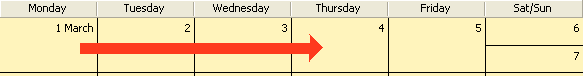The first part of the formula gives the days. (4 – 1) gives three whole days. The second part of the formula gives the time. 10am – 9pm gives 13 hours. But that gives three days and thirteen hours, whereas it is really two whole days and thirteen hours. The formula therefore needs to subtract twenty-four hours from the total if the second time is before the first. To do this, you test to see if the second time is before the first using the logical expression `(TimeB<TimeA)`. By using the num keyword, you can turn the result of the test into a numeric value, so it will be 1 for true and 0 for false. If you multiply this result by 24, you get the right number of hours to take away, 0 if TimeB isn’t smaller than TimeA, and 24 if it is.`(24 *num(TimeB<TimeA))`So the whole formula reads:`(DateB-DateA)*24 + (TimeB-TimeA)/3600 - 24*num(TimeB<TimeA)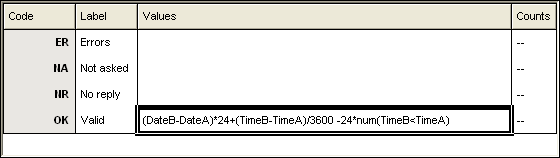`
9. Press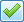or click  [Enter] to save your new variable.

### Step 4: Splitting up the data into bands for analysis

1. Clickto create a new variable to show the length of the journey.
2. Set the Name to tripType, the Type to Derived, and the Response to Single. Add a label describing the variable function
3. In the first Label field type Quick trip, and for its value type timedelay<=2. All cases where the time taken is left than or equal to two hours will be stored as quick trips.
4. In the second Label field type Medium trip and type timedelay <=6 as the value. All cases where the time taken is 2 to 6 hours will be stored here.
5. In the third Label field type Long trip and type timedelay >6 as its values. All cases where the time taken is greater than six hours are stored as Long trips.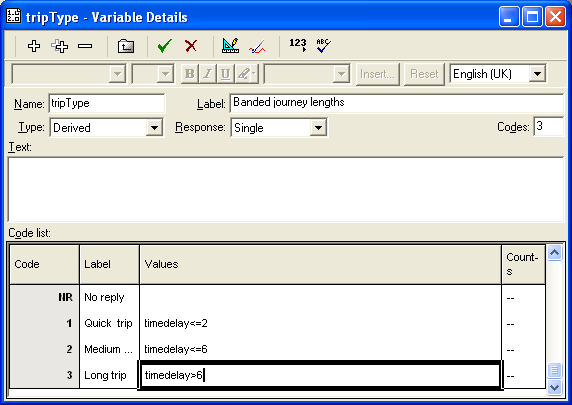You can now perform analyses using either the tripType or the timedelay variable. For example: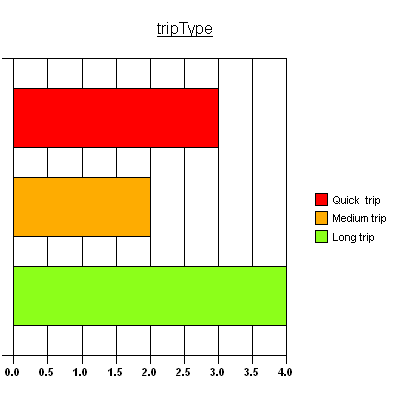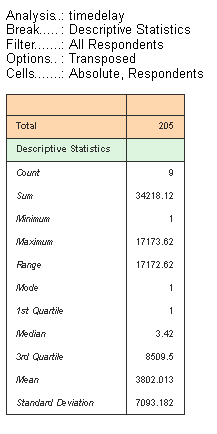### Changing the variable names back to question numbers

When you changed the variable names to DateA and DateB et cetera, it will have confused the question numbering in your survey. You can change them back to Q1.a, Q2.a et cetera, and the variable names will automatically be changed in the formula for timedelay.

Alternatively, you can hide the question numbers by selecting the questions, the choosing Show in the toolbar topics, Name in the dropdown list, and clearing the Show box.If there is a topic you would like a worksheet on, email to snapideas@snapsurveys.com